# Periodic Classification of Elements Class 10 Extra Questions with Answers Science Chapter 5

In this page, we are providing Periodic Classification of Elements Class 10 Extra Questions and Answers Science Chapter 5 pdf download. NCERT Extra Questions for Class 10 Science Chapter 5 Periodic Classification of Elements with Answers will help to score more marks in your CBSE Board Exams.

## Class 10 Science Chapter 5 Extra Questions and Answers Periodic Classification of Elements

Extra Questions for Class 10 Science Chapter 5 Periodic Classification of Elements with Answers Solutions

### Extra Questions for Class 10 Science Chapter 5 Very Short Answer Type

Question 1.
Name two elements whose properties were predicted on the basis of their positions in Mendeleev’s periodic table.
Gallium (Eka-aluminium) and germanium (Eka-silicon).

Question 2.
Write the formulae of chlorides of Eka-silicon and Eka-aluminium, the elements predicted by Mendeleev.  [NCERT Exemplar]
Eka-aluminium represents gallium (Ga) with valency three and Eka-silicon is for germanium (Ge) with valency four. The formulae of their respective chlorides are GaCl3 and GeCl4.

Question 3.
How many elements are there in period 2?
Eight (8).

Question 4.
Name all the elements present in group-17 of the Modern Periodic Table.
F, Cl, Br, I, At.

Question 5.
Name two elements whose atomic weight were corrected on the basis of their position in Mendeleev’s periodic table.
Gold (Au) and platinum (Pt).

Question 6.
Arrange the following elements in the increasing order of their metallic character.
Mg, Ca, K, Ge, Ga     [NCERT Exemplar]
Ge < Ga Mg < Ca < K.

Question 7.
Name the scientist who proposed the Modern Periodic Law.
Henry Moseley, a scientist, proposed the Modern Periodic Law.

Question 8.
What is meant by a group in the periodic table?
Groups are the vertical columns/vertical lines in the periodic table.

Question 9.
What is meant by a period in the periodic table?
Periods are the horizontal rows in the periodic table.

Question 10.
Name the elements present in period 1 of the modern periodic table.
Hydrogen (H) and Helium (He).

Question 11.
An element A is in group II (group 2) of the periodic table:
(а) What will be the formula of its chloride?
(b) What will be the formula of its oxide?
(a) ACl2
(b) AO.

Question 12.
Why are the elements calcium, strontium and barium named as alkaline earths?
These elements are called alkaline earths because their oxides are alkaline in nature and exist in the earth.

Question 13.
Give the name and electronic configuration of second alkali metal.
The second alkali metal is sodium (Na). Its electronic configuration is 2, 8, 1.

Question 14.
What are Metalloids?
Those elements which show some properties of both metals and non-metals are called metalloids. Examples: Boron, Silicon, Germanium, Arsenic, Antimony, Tellurium and Polonium.

Question 15.
Name three elements whose atomic masses were correct on the basis of their positions in Mendeleev’s periodic table.

1. Beryllium (Be, group II A)
2. gold (Au, group II B)
3. platinum (Pt, group VIII).

Question 16.
Name three elements which behave as metalloids.
The elements are:

1. arsenic (As)
2. antimony (Sb)
3. germanium (Ge).

Question 17.
Name the inert gas which has two electrons in its valence shell.
Helium.

Question 18.
Name the most metallic and most non-metallic element in the Periodic Table.
The most metallic elements is francium (group 1) and most non-metallic element is fluorine (group 17).

Question 19.
In the Modern Periodic Table, which are the metals among the first ten elements?
Lithium (Li), Beryllium (Be) and Boron (B) are the metal elements among first ten elements in the Modern Periodic Table.

Question 20.
Two elements A and B belong to the same period. What is common in them?
They have the same number of shells.

Question 21.
The electronic configuration of an element is 2, 8, 6. Identify the element and name of the family to which it belongs.
The element with configuration 2, 8, 6 (Z = 16) is sulphur. It belongs to the oxygen family.

Question 22.
Calcium, Strontium and Barium form a Dobereiner’s triad. The atomic masses of calcium and Barium are 40 and 137 respectively. Predict the atomic mass of strontium.
According to Dobereiner’s triad, atomic mass of strontium is the arithmetic means of Ca and Ba as strontium lies between Ca and Ba.
Atomic mass of Sr = $$\frac{40+137}{2}=\frac{177}{2}=88.5$$

Question 23.
The formula of magnesium oxides is MgO. Write the formula of magnesium chloride.
Oxygen is divalent in nature. The valency of magnesium in magnesium oxide is + 2. The formula of magnesium chloride is MgCl2 since chlorine has valency equal to one.

Question 24.
State whether the following statement is true or false?
The valency of an element of group 17 is 7.
This statement is wrong. The number of valence electrons in an element of group 17 = 17 – 10 = 7.
Therefore, the valency of the element = 8 – 7 = 1.

Question 25.
Name two elements whose atomic weight were corrected on the basis of their position in Mendeleev periodic table.

1. Gold (Au)
2. Platinum (Pt).

Question 26.
The two isotopes of chlorine have atomic mass 35.4 and 37.4. Should they be placed in separate slots in the modern periodic table?
No, they should be placed in the same slot because the modern periodic table is based on the atomic number of the elements.

Question 27.
List any two properties of the elements belonging to the first group of the modem periodic table.   [Delhi 2014]
Elements of first group have one valence electron each in their atoms. All the elements of group 1 have the same valency of 1.

Question 28.
What is the basic difference in the electronic configuration of the elements belonging to group 1 and group 2?
All elements belonging to group 1 have one electron in the valence shell while all elements belonging to group 2 have two electrons in their valence shell.

Question 29.
Element ‘Y’ with atomic number 3 combines with element ‘A’ with atomic number 17. What will be the formula of the compound?
The electronic distribution in elements ‘A’ and ‘Y’ are 2, 1 and 2, 8, 7 respectively. Both have valency equal to 1. The formula of the compound is AY.

Question 30.
Name the property which remains unchanged on descending a group in the periodic table.
Valence electrons.

Question 31.
An element X is in group 13 of the periodic table. What is the formula of its oxide?
X2O3.

Question 32.
Elements ‘X’ and TP belong to groups 1 and 17 of the periodic table respectively. What will be the nature of the bond in the compound XY?
Ionic bond.

### Extra Questions for Class 10 Science Chapter 5 Short Answer Type I

Question 1.
The three elements A, B and C with similar properties have atomic masses X, Y and Z respectively. The mass of Y is approximately equal to the average mass of X and Z. What is such an arrangement of elements called? Give one example of such a set of elements.   [NCERT Exemplar]
The arrangement of these elements is known as Dobereiner triad. Example, Lithium, sodium and Potassium.

Question 2.
In group I of the periodic table, three elements X, Y and Z have atomic radii 1.33 A, 0.95 A and 0.60 A respectively. Arrange the elements (X, Y and Z) in the increasing order of atomic number and mention a suitable reason for it.
In a given group, the atomic radii increases on moving down the group. Therefore, the arrangement of the given elements in the increasing order of their atomic numbers is as follows: Z, Y, X.

Question 3.
Elements have been arranged in the following sequence on the basis of their increasing atomic masses.
F, Na, Mg, Al, Si, P, S, Cl, Ar, K
(a) Pick two sets of elements which have similar properties.
(b) The given sequence represents which law of classification of elements?   [NCERT Exemplar]
(a) F and Cl are first and eighth element in the above sequence, therefore, they have similar properties.
Please note that although Na and K have similar properties but they are not related as first and eighth element in the above sequence.

(b) This sequence represents Newland’s Law of Octaves.

Question 4.
State and explain Mendeleev’s periodic law.
According to Mendeleev’s periodic law “Physical and chemical properties of elements are periodic function of their atomic masses”.

If elements are arranged in the increasing order of atomic masses, elements with similar properties lies after a certain interval in the periodic table.

Question 5.
“Hydrogen occupies a unique position in the Modern Periodic Table”. Justify the statement.   [NCERT Exemplar]
The position of the element hydrogen is still not clear even in the Modern Periodic Table.

In electronic configuration, it resembles alkali metals of group 1. All of them have only one electron in the valence shell. Actually, hydrogen has only one shell (K-shell) which has one electron.

In characteristics, it resembles halogens of group 17. For example, like halogens hydrogen is a non-metal and diatomic as well. It has been therefore, decided to assign hydrogen a unique position in the Modern or Long Form Periodic Table. It is placed at the top in group 1 of alkali metals. However, it is not a member of that group.

Question 6.
If an element X is placed in group 14, what will be the formula and the nature of bonding of its chloride?  [NCERT Exemplar]
Since element “X’ is placed in group 14, therefore, its valency is 14 – 10 = 4. Further, since it is difficult to either lose all the four valence electrons or gain four more electrons, therefore, it prefers to share these four electrons to acquire the stable electronic configuration of the nearest inert gas. Its formula will be XCl4. Thus, the nature of the chloride of element ‘X’ is covalent.

Question 7.
Explain clearly, why atomic number of an element is most important to the chemist than its relative atomic mass.
Atomic number corresponds to the number of electron in an atom or it reflects the electronic configuration of the element. The elements having similar electronic configurations can then be placed together in the same group, it helps in the systematic classification of elements.

On the other hand, the atomic mass of an element does not provide the electronic configuration of an element, so atomic number is more fundamental property for classification of elements.

Question 8.
What is the need of classification of the elements?
The following reasons can be assigned for practical utility of classification of elements:

• To make the study of chemical elements easier.
• To correct atomic masses of various elements.
• To discover new elements.

Question 9.
The elements calcium, strontium and barium were put in one group or family on the basis of their similar properties.
(i) Mention the two similar properties?
(ii) What is the usual name of this group or family?
(i) (a) All these elements are metals.
(b) All these elements have a valency of 2.

(ii) The usual name of this group or family is alkaline earth metals.

Question 10.
How does the electronic configuration of an atom of an element relate to its position in the modem periodic table? Explain with one example.   [CBSE 2011 (Delhi)]
The electronic configuration of an atom of an element gives its position in the modern periodic table.
(i) The ‘period number’ of an element is equal to the number of electron shells in its atom.
(ii)

• The group number of an element having upto two valence electrons is equal to the number of valence electrons.
• The group number of an element having more than 2 valence electrons is equal to the number of valence electrons plus 10.

Example: If the electronic configuration of an element is 2, 8, 7.
Then its period number is 3 as it has three electrons shells.
Its group number is 17 as it has 7 valence electrons. (∵ Group no. = 7 + 10 = 17)

Question 11.
An element X (atomic number 17) combines with an element Y (atomic number 20) to form a compound.
(i) Write the positions of these elements in the modern periodic table.
(ii) Write the formula of the compound formed. Justify your answer in each case.   [CBSE 2013]
(i) The electronic configurations of the two elements are:
X (Z = 17) 2, 8, 7 ; Y (Z = 20) 2, 8, 8, 2.
Element X is present in group 17 (halogen family) since it has 7 valence electrons. Element Y is placed in group 2 (alkaline earth family) since it has 2 valence electrons.

(ii) Element Y is a metal with valency 2 while element X is a non-metal with valency 1. Therefore, they two combine to form compound YX2.

Question 12.
Explain why:
(i) All the elements in a group have similar chemical properties.
(ii) All the elements in a period have different chemical properties.
(i) All the elements in a particular group have similar outer shell electronic configuration. Since chemical properties of an element are determined mainly by the outer shell configuration, all the elements in a group have similar chemical properties.

(ii) All the elements in a period have different valence shell electronic configuration because they have different number of electrons in the valence shell. Hence, the elements in a period have different chemical properties.

Question 13.
Given below are the atomic radii of three elements X, Y and Z of the periodic table, each having n electrons in the outermost shell of their atoms:

 Element X Y Z Atomic radii 133 pm 157 pm 202 pm

(a) Do these elements X, Y and Z belong to same group or to the same period?
(b) Which element will be least metallic?
(a) Since the elements X, Y and Z contain same number of electrons (n) in the valence shell (outermost shell), they belong to the same group.
(b) In a group, the atomic radius increases on descending the group. Therefore, the element X with the smallest atomic radius is at the top of the group and the element Z having largest atomic radius is at the bottom.

Now, we know metallic character increases as we move from top to bottom in a group. Therefore, the least metallic element is X.

Question 14.
(a) The elements of the second period along with their atomic numbers in parentheses are given below:
B (5), Be (4), O (8), N (7), Li (3), C (6), F (9)
(i) Arrange them in the same order as they appear in the periodic table.
(ii) Which element has the largest and smallest atom?
(b) Why does atomic radius change as we move from left to right in a period?   [CBSE 2011]
(a) (i) Li (3), Be (4), B (5), C (6), N (7), O (8), F (9)
(ii) The element Li has the largest atom. The element F has the smallest atom.

(b) Along a period, the nuclear charge increases and the electrons are attracted more towards the nucleus. Therefore, the atomic size or atomic radius decreases as we move from left to the right along a period.

Question 15.
Write two reasons responsible for the late discovery of noble gases.   [CBSE 2013]

1. Noble gas elements were not present in earth crust as minerals like other elements and were present in air to a very small extent.
2. Their atoms have stable electronic configuration of their outermost shells also called valence shells. (2 in case of He and 8 for other elements). They do not combine with atoms of other elements.

That is why, noble gas elements were discovered at a later stage.

Question 16.
From the following elements:
4Be; 9F; 19K; 20Ca
(i) Select the element having one electron in the outermost shell.
(ii) two elements of the same group.
Write the formula of and mention the nature of the compound formed by the union of 19K and element X (2, 8, 7). [Delhi 2015]
(i) 19K
(ii) 4Be, 20Ca belong to the same group.
Electronic configuration of K (19) = 2, 8, 8, 1   Valency of K = 1
Electronic configuration of X (2, 8, 7)   Valency of X = 1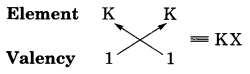The formula of the compound formed is KX. The compound KX is of ionic nature.
The bond is formed by transference of electrons.

Question 17.
Na, Mg and Al are the elements of the same period of Modern Periodic Table having one, two and three valence electrons respectively. Which of these elements (i) has the largest atomic radius, (ii) is least reactive? Justify your answer stating reason for each case.   [Delhi 2015]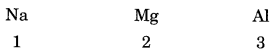(1) Na has the largest atomic radius because on moving from left to right in the periodic table, the atomic radius decreases due to increase in positive charge on the nucleus which pulls the outermost electrons more close to the nucleus and the size of atom decreases.

(2) Al is least reactive because on moving from left to right in the periodic table the nuclear charge increases and the valence electrons are pulled in more close to the nucleus. Therefore, the tendency to lose electrons decreases and hence reactivity decreases.

Question 18.
How many groups and periods are there in the modern periodic table? How do the atomic size and metallic character of elements vary as we move: (a) down a group and (b) from left to right in a period. [Delhi 2015]
Number of groups is 18 and number of periods in the modern periodic table is 7.
(a) All the elements in a group have the same valency. On going down in a group, the atomic size increases because a new shell of electrons is added to the atoms at every step.

(b) On moving from left to right in the periodic table, the valency of the elements first increases from 1 to 4 and then decreases to zero. On moving from left to right in a period, the metallic character of elements decrease because on moving from left to right in a period, the electropositive character of elements decrease.

Question 19.
The electronic configuration of an element X is: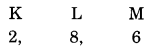(i) What is the group number of element X in the periodic table?
(ii) What is the period number of element X in the periodic table?
(iii) What is the number of valence electrons in an atom of X?
(iv) What is the valency of X?
(i) From the above given electronic configuration we find that element X has 6 valence electrons in the outermost shell), so the group number of element X in the periodic table is 6 + 10 = 16.

(ii) Element X has 3 electron shells (K, L and M) in its atom, so the period number of X is 3. That is, X belongs to 3rd period of the periodic table.

(iii) Element X has 6 valence electrons.

(iv) Element X has 6 valence electrons so it needs 2 more electrons to complete its octet (8 electrons in valence shell) and become stable. Thus, the valency of element X is 2.

### Extra Questions for Class 10 Science Chapter 5 Short Answer Type II

Question 1.
Identify the elements with the following property and arrange them in increasing order of their reactivity.
(a) An element which is a soft and reactive metal.
(b) The metal which is an important constituent of limestone.
(c) The metal which exists in liquid state at room temperature.  [NCERT Exemplar]
(a) Alkali metals are soft and reactive. For example, Na, K, etc.
(b) Limestone is calcium carbonate, therefore, the important constituent of limestone is calcium.
(c) Metals which exists in the liquid state at room temperature are Mercury (Hg).

Question 2.
List three main anomalies of Mendeleev’s classification of elements.
Anomalies or defects of Mendeleev’s periodic classification of elements are as follows:

1. Position of hydrogen.
2. Position of isotopes: Isotopes of an element were not assigned separate places.
3. Elements which are chemically similar such as gold and platinum have been placed in separate groups.

Question 3.
(a) State modern periodic law.
(b) What are the advantages of the long form of the periodic table over Mendeleev’s periodic table?
(a) According to modern periodic law “physical and chemical properties of elements are periodic function of their atomic numbers”.

(b) (i) Classification of elements on the basis of atomic number is more closer to chemical properties.
(ii) No separate places for isotopes of an element are required.
(iii) In case of Ar and K, Ar has less atomic number so it should be placed before K according to the increasing order of atomic number.

Question 4.
Two elements X and Y have atomic number 12 and 17 respectively.
(i) Write the electronic configuration of both.
(ii) Which type of bond will they form?
(iii) Write the formula of the compound formed by their combination (in terms of X and Y).
(i) The electronic configuration of element X (Z = 12) = 2, 8, 2
The electronic configuration of element Y (Z = 17) = 2, 8, 7

(ii) The bond formed will be of ionic nature. One atom of X will transfer 1 electron each to two atoms of Y.

(iii) The formula of the compound is: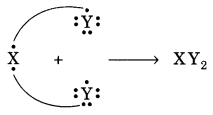Question 5.
Name:
(a) Three elements that have a single electron in their outermost shells.
(b) Two elements that have two electrons in their outermost shells.
(c) Three elements with filled outermost shells.
(a) Lithium (Li), Sodium (Na), Potassium (K)
(b) Magnesium (Mg), Calcium (Ca)
(c) Helium (He), Neon (Ne), Argon (Ar)

Question 6.
Two elements X and Y belong to group 1 and 2 respectively in the same period of periodic table. Compare them with respect to:   [CBSE 2011]
(i) the number of valence electrons in their atoms
(ii) their valencies
(iii) metallic character
(iv) the sizes of their atoms
(v) the formulae of their oxides
(vi) the formulae of their chlorides.
X and Y belong to same period.

• X belongs to group ‘1’.
• Y belongs to group ‘2’.

(i) Valence electron in X is 1 whereas valence electrons in Y are 2.
(ii) The valency of X is 1 whereas valency of Y is 2.
(iii) X is more metallic than Y because metallic character decreases on moving from left to right in a period.
(iv) The size of X is more than Y because size of the atom decreases on moving from left to right in a period.
(v) Oxide of X = X2O,   Oxide of Y = YO
(vi) Chloride of X = XCl,   Chloride of Y = YCl2

Question 7.
The element Li, Na and K, each having one valence electron, are in period 2, 3 and 4 respectively of the Modem Periodic Table.
(a) In which group of the periodic table should they be?
(b) Which one of them is least reactive?
(c) Which one of them has the largest atomic radius? Give reason to justify your answer in each case. [CBSE 2013]
(a) Since the elements have one valence electron, they are placed in group 1 (Alkali metals) in the order Li (period = 2); Na (period = 3); K (period = 4).

(b) Since the reactivity of the elements increases down a group, the element Li is the least reactive chemically.

(c) Since the atomic size increases down a group, the element K has the large atomic size or atomic radius out of these elements.

Question 8.
Explain why are the following statements not correct:
(a) All groups contain metals and non-metals.
(b) Atoms of elements in the same group have the same number of electron(s).
(c) Non-metallic character decreases across a period with increase in atomic number.
(d) Reactivity increases with atomic number in a group as well as in a period.   
(a) Because all groups do not contain metals and non-metals. For example, Alkali metal group contains metals only.
(b) Atom of the elements in the same group have same number of electrons in the valence shell.
(c) Non-metallic character increases across a period with increase in atomic number.
(d) Reactivity increases with atomic number in a group but not across a period.

Question 9.
This question refers to the elements of the periodic table with atomic numbers from 3 to 18. Some of the elements are shown by letters but the letters are not the usual symbols of the elements.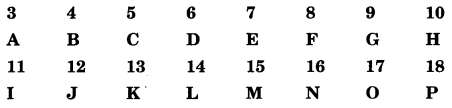(a) Which of these
(i) are noble gases?
(ii) are halogens?
(iii) are alkali metals?
(b) If A combines with F, what would be the formula of the resulting compound?
(c) What is the electronic configuration of G?
(a) (i) H, P;
(ii) G, O;
(iii) A, I
(b) F
(c) 2, 7

Question 10.
Name two other elements which are in the same group as:
(i) Carbon
(ii) Fluorine
(iii) Sodium, respectively.
(i) The two other elements which are in the same group as carbon are: Silicon (Si) and germanium (Ge).
(ii) The two other elements which are in the same group as fluorine are : Chlorine (Cl) and bromine (Br).
(iii) The two other elements which are in the same group as sodium are : Lithium (Li) and potassium (K).

Question 11.
Atomic number of an element is 16. Predict
(i) the number of valence electrons in its atom
(ii) its valency
(iii) its group number
(iv) whether it is a metal or a non-metal
(v) the nature of oxide formed by it.
(vi) the formula of the chloride   [CBSE2011]
The element with atomic number Z = 16 is sulphur. The electronic configuration of the element is 2, 8, 6.
(i) The number of valence electrons is 6
(ii) The valency of the element is 2 (8 – 6 = 2)
(iii) The group number of the element is 16.
(iv) The element is a non-metal.
(v) Since the element is non-metal, its oxide is acidic in nature. Actually, two oxides are formed. These are SO2 and SO3. Both are of acidic nature.
(vi) The formula of the chloride is SCl2.

Question 12.
(a) How are the following related?
(i) Number of valence electrons of the different elements present in the same group.
(ii) Number of the shells of the elements in the same period.
(b) How do the following change:
(i) Number of shells of the elements as we go down a group.
(ii) Number of valence electrons of elements on moving from left to right in a period.
(iii) Atomic radius in moving from left to right along a period.
(iv) Atomic size down a group. [CBSE 2012]
(a) (i) Number of valence electrons of the elements present in a group do not change.
(ii) The elements present in the same period have same number of shells.

(b) (i) The number of shells of elements in a group gradually increase downwards.
(ii) The number of valence electrons of the elements in moving from left to right along a period gradually increase.
(iii) Atomic radius decreases from left to the right.
(iv) Atomic size increases down a group.

Question 13.
(a) What is meant by periodicity in properties of elements with reference to the periodic table?
(b) Why do all the elements of the same group have similar properties?
(c) How will the tendency to gain electrons change as we go from left to right across a period? Why?
(a) Periodicity in properties of elements with reference to the periodic table means the elements having similar properties are repeated after certain intervals or periods and the elements are arranged in the tabular form.

(b) All the elements of the same group have similar properties because all the elements belonging to the same group of the Periodic Table have same number of valence electrons.

(c) On moving from left to right in a period, the tendency of atoms to gain electrons increases because on moving from left to right in a period the nuclear charge (positive charge on nucleus) increases due to gradual increase in the number of protons so it becomes easier for the atoms to gain electrons.

Question 14.
What is meant by ‘group’ in the modern periodic table? How do the following change on moving from top to bottom in a group?
(i) Number of valence electrons
(ii) Number of occupied shells
(iii) Size of atoms
(iv) Metallic character of elements
(v) Effective nuclear change experienced by valence electrons.  [CBSE 2014]
The vertical columns in a periodic table are called groups.
(i) All the elements of a group have the same number of valence electrons.
(ii) On moving down in a group the number of occupied (filled) shells increases gradually.
(iii) On going down in a group the size of atoms increases because a new shell of electrons is added to the atoms at every step.
(iv) On going down in a group the metallic character of elements increases.
(v) On moving down in a group, one more electron shell is added at every stage and size of the atom increases. Thus valence electrons move more and more away from the nucleus and hold of the nucleus or nuclear charge on valence electrons decreases.

Question 15.
The elements Be, Mg and Ca each having two electrons in their outermost shells are in periods 2, 3, and 4 respectively of the modern periodic table. Answer the following questions, giving justification in each case:  [CBSE 2014]
(i) Write the group to which these elements belong.
(ii) Name the least reactive element.
(iii) Name the element having largest atomic radius.

 Element Valence electrons Period Electronic configuration Be 2 2 2,2 Mg 2 3 2, 8, 2 Ca 2 4 2, 8, 8, 2

(i) All these elements belong to the 2nd group all have two electrons in their outermost shell.

(ii) Be is the least reactive metal because reactivity of metals increases in a period as the tendency to lose electrons in a group increases.

Therefore, Be being the smallest in all the given elements of a period has its valance electrons nearest to the nucleus. So the removal of electrons from its valance shell will be difficult.

(iii) Ca has the largest atomic radius because it has maximum number of shells, i.e., 4.

Question 16.
An element ‘M’ with electronic configuration (2, 8, 2) combines separately with (NO3), (SO4)2- and (PO4)3- radicals. Write the formula of three compounds so formed. To which group and period of the Modern Periodic Table does the elements ‘M’ belong? Will ‘M’ from covalent or ionic compounds? Give reason to justify your answer.
Element M with electronic configuration =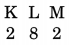Total number of electrons = 2 + 8 + 2 = 12, i.e., the given element is Magnesium (Mg). Formula of compound formed are: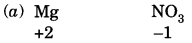Formula of compound = Mg (NO3)2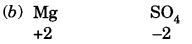Formula of compound = MgSO4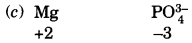Formula of compound = Mg3 (PO4)2

M will form ionic compound with radicals given. An ionic compound is a chemical compound comprising ions held together by electrostatic forces. In this compound no sharing of electrons takes place.

### Extra Questions for Class 10 Science Chapter 5 Long Answer Type

Question 1.
An element X which is a yellow solid at room temperature shows catenation and allotropy. X forms two oxides which are also formed during the thermal decomposition of ferrous sulphate crystals and are the major air pollutants.
(a) Identify the element X
(b) Write the electronic configuration of X
(c) Write the balanced chemical equation for the thermal decomposition of ferrous sulphate crystals.
(d) What would be the nature (acidic/basic) of oxides formed?
(e) Locate the position of the element in the Modern Periodic Table.  [NCERT Exemplar]
(a) The element X which is a yellow solid at room temperature and shows catenation and allotropy is sulphur (S).

(b) The atomic number of sulphur is 16. Therefore, its electronic configuration is 2, 8, 6.

(c)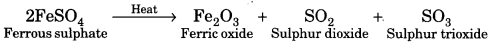Both SO2 and SO3 are major air pollutants.

(d) Since sulphur is a non-metal, therefore, both SO2 and SO3 are acidic oxides since they dissolve in water to form the corresponding acids.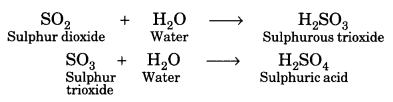(e) Since sulphur contains six valence electrons, therefore, it lies in group 6 + 10 = 16. Further, since atomic number of sulphur (S) is 16, it lies in the 3rd period.

Question 2.
An element X (atomic number 17) reacts with an element Y (atomic number 20) to form a divalent halide.
(a) Where in the periodic table are elements X and Y placed?
(b) Classify X and Y as metal, non-metal or metalloid.
(c) What will be the nature of oxide of element Y? Identify the nature of bonding in the compound formed.
(d) Draw the electron dot structure of the divalent halide.   [NCERT Exemplar]
(a) X belongs to Group 17 and 3rd Period.
Y belongs to Group 2 and 4th Period.
(b) X-non-metal and Y-metal.
(c) Basic oxide; Ionic bonding.
(d)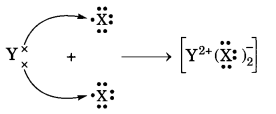Question 3.
(a) Why do we classify elements?
(b) What were the two criteria used by Mendeleev in creating his Periodic Table?
(c) Why did Mendeleev leave some gaps in his Periodic Table?
(d) In Mendeleev’s Periodic Table, why was there no mention of Noble gases like Helium, Neon and Argon?
(e) Would you place the two isotopes of chlorine, Cl-35 and Cl-37 in different slots because of their different atomic masses or in the same slot because their chemical properties are the same? Justify your answer.
(a) As different elements were being discovered, scientists gathered more information about the properties of these elements. It was observed that it was difficult to organise all the information or properties of these elements. So scientists started discovering some pattern in their properties to classify all the known elements to make their study easier.

(b) Atomic mass and similarity of chemical properties (compounds formed by elements with oxygen and hydrogen) were the two criteria used by Mendeleev in his Periodic Table.

(c) Mendeleev left some gaps in his Periodic Table as he predicted the existence of some elements that had not been discovered at that time.

(d) Noble gases like helium, neon, argon, etc. were not mentioned in Mendeleev’s Periodic Table because these gases were discovered later as they are very inert and present in extremely low concentrations in our atmosphere. After the discovery of noble gases they could be placed in a new group without disturbing the existing order of the Periodic Table.

(e) The Modem Periodic Table states that properties of elements are a periodic function of their atomic numbers, since Cl-35 and Cl-37 isotopes have the same atomic number (17), hence they will have same chemical properties even though their atomic masses are different. So, they should be placed in the same slot of the periodic table.

Question 4.
Atomic number of a few elements are give below:
10, 20, 7, 14
(a) Identify the elements.
(b) Identify the Group number of these elements in the Periodic Table.
(c) Identify the Periods of these elements in the Periodic Table.
(d) What would be the electronic configuration for each of these elements?
(e) Determine the valency of these elements.  [NCERT Exemplar]
(a) The element are: Neon (Z = 10), Calcium (Z = 20), Nitrogen (Z = 7) and Silicon (Z = 14).
(b) Group number: Neon (18), Calcium (2), Nitrogen (15), Silicon (14).
(c) Periods: Neon (2), Calcium (4), Fluorine (2), Silicon (3).
(d) Electronic Configuration: Neon (2, 8); Calcium (2, 8, 8, 2); Nitrogen (2, 5); Silicon (2, 8, 4).
(e) Valency: Neon (zero); Calcium (2); Nitrogen (3); Silicon (4).

Question 5.
This question refers to the elements of the periodic table with atomic numbers from 3 to 18. Some of the elements are shown by letters, but the letters are not the usual symbols of the elements.

 3 4 5 6 7 8 9 10 A E G 11 12 13 14 15 16 17 18 B C D F

(a) Which of these:
(i) is a noble gas?
(ii) is a halogen?
(iii) is an alkali metal?
(iv) is an element with valency 4?
(b) If A combines with F, what would be the formula of the resulting compound?
(c) What is the electronic arrangement of G?
(a) (i) G having 8 electrons in its valence shell is a noble gas.
10G : 2, 8

(ii) F having 7 electrons in its valence shell is a halogen.
17F : 2, 8, 7

(iii) B having 1 electron in its valence shell is an alkali metal.
11B : 2, 8, 1
Element A is also an alkali metal.
3A : 2, 1

(iv) The element D having 4 electrons in its valence shell will have valency 4.
14D : 2, 8, 4

(b) A is an electropositive element with valency 1 while F is an electronegative element with valency 1.
Hence, the formula of the compound between A and F would be AF.

(c) The electronic configuration of G is:
10G : 2, 8.

Question 6.
The elements of one short period of the periodic table are given below in the order from left to right.
Li, Be, B, C, O, F, Ne,
(a) To which period these elements belong?
(b) One element of this period is missing. Which is the missing element and where should it be placed?
(c) Which one of the element in this period shows the property of catenation?
(d) Place the three elements fluorine, beryllium and nitrogen in the order of increasing electronegativity.
(e) Which one of the above elements belong to the halogen series?
(a) Second period (Atomic number: 3-10)
(b) Nitrogen (N), It should be placed between Carbon (Atomic number 6) and Oxygen (Atomic number 8)
(c) Carbon
(d) Be, N, F
(e) Fluorine.

Question 7.
Which element has
(a) two shells, both of which are completely filled with electrons?
(b) the electronic configuration 2, 8, 2?
(c) a total of three shells, with four electrons in its valence shell?
(d) a total of two shells, with three electrons in its valence shell?
(e) twice as many electrons in its second shell as in its first shell?
(a) Neon [Ne] (2, 8)
(b) Magnesium [Mg]
(c) Silicon [Si] (2, 8, 4)
(d) Boron [B] (2, 3)
(e) Carbon [C] (2, 4).

### Periodic Classification of Elements HOTS Questions With Answers

Question 1.
(a) In this ladder symbols of elements are jumbled up. Rearrange these symbols of elements in the increasing order of their atomic number in the Periodic Table.
(b) Arrange them in the order of their group also.  [NCERT Exemplar]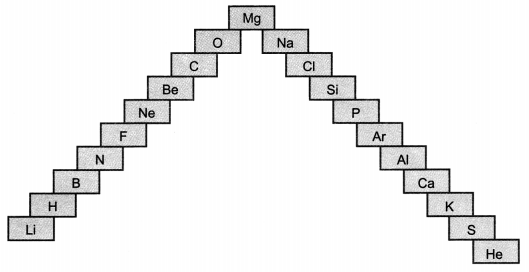(a) H, He, Li, Be, B, C, N, O, F, Ne, Na, Mg, Al, Si, P, S, Cl, Ar, K, Ca
(b) Group 1 – H, Li, Na, K
Group 2 – Be, Mg, Ca
Group 13 – B, Al
Group 14 – C, Si
Group 15 – N, P
Group 16 – O, S
Group 17 – F, Cl
Group 18 – He, Ne, Ar.

Question 2.
The electronic configuration of an element T is 2, 8, 7.
(а) What is the group number of T?
(b) What is the period number of T?
(c) How many valence electrons are there in an atom of T?
(d) Is it a metal or non-metal?
(a) Group-17
(b) Third period
(c) 7
(d) Non-metal.

Question 3.
Mendeleev predicted the existence of certain elements not known at that time and named two of them as Eka-silicon and Eka-aluminium.
(a) Name the elements which have taken the place of these elements.
(b) Mention the group and the period of these elements in the Modern Periodic Table.
(c) Classify these elements as metals, non-metals or metalloids.
(d) How many valence electrons are present in each one of them?  [NCERT Exemplar]
(a) Eka-silicon for germanium (Ge) and Eka-aluminium for Gallium (Ga).
(b) Group number of Ga is 13 and its period is 4th and group number of Ge is 14 and its period is also 4th.
(c) Both Ga and Ge are metalloids.
(d) Ga lies in group 13, therefore, it has 13 – 10 = 3 valence electrons. Similarly, Ge lies in group 14 and hence it has 14 – 10 = 4 valence electron.

Question 4.
The atomic numbers of three elements A, B and C are given below:

 Element Atomic number A 5 B 7 C 10

(i) Which element belongs to group-18?
(ii) Which element belongs to group-5?
(iii) Which element belongs to group-3?
(iv) To which period/periods do these elements belong?
(i) C
(ii) B
(iii) A
(iv) Second period.

Question 5.
(a) Electropositive nature of the element(s) increases down the group and decreases across the period.
(b) Electronegativity of the element decreases down the group and increases across the period.
(c) Atomic size increases down the group and decreases across a period (left to right).
(d) Metallic character increases down the group and decreases across a period.
On the basis of the above trends of the Periodic Table, answer the following about the elements with atomic numbers 3 to 9.
(a) Name the most electropositive element among them
(b) Name the most electronegative element
(c) Name the element with smallest atomic size
(d) Name the element which is a metalloid
(e) Name the element which shows maximum valency.  [NCERT Exemplar]
(a) Lithium (Z = 3) is the most electropositive element.
(b) Fluorine (Z = 9) is the most electronegative element.
(c) Fluorine (Z = 9) has the smallest atomic size.
(d) Boron (Z = 5) is a metalloid.
(e) Carbon (Z = 6) shows the maximum valency (4).
However, the element nitrogen (Z = 7) can show valency 5 in some compounds (example, N2O5).

Question 6.
The following table shows the position of six elements A, B, C, D, E and F in the period table.

 Groups/periods 1 2 3 to 12 13 14 15 16 17 18 2. A B C 3. D E F

Using the above table answer the following questions:
(a) Which element will form only covalent compounds?
(b) Which element is a metal with valency of 2?
(c ) Which element is a non-metal with valency of 3?
(d) Out of D and E, which one has more atomic radius and why?
(e) Write a common name for the family of elements C and F.
(a) The element ‘E’ present in group 14 is a non-metal. Its name is silicon (Si) and the compounds of the element are only covalent.
(b) The element ‘D’ present in group 2 is a metal known as magnesium (Mg). It exhibits valency 2 in its compounds.(c) The elements ‘B’ present in group 15 is a non-metal. It is nitrogen (N) and exhibits valency 3 in its compounds.
(d) The element ‘D’ has more atomic radius than the element ‘E’ as the atomic size decreases along a period.
(e) The elements ‘C’ and ‘F’ present in group 18 belong to a family known as noble gases.

Question 7.
From the part of the periodic table given, answer the following questions

 1 Lithium 2 13 14 Carbon 15 16 Oxygen 17 L 18 Neon X S P Q Y R Z T

(a) Which is the most reactive metal?
(b) Name the family of L, Q, R and T.
(c) Name one element of group 2 and 15.
(d) Name one member of group 18 other than neon.
(e) Give the name of the element S placed below carbon in group 14.
(a) Z
(b) L, Q, R and T belongs to halogen family.
(c) Group-2 element – Ca, Mg
Group-15 element – N, P
(d) The element argon (Ar) is also present in group 18.
(e) The element is silicon (Si).

### Extra Questions for Class 10 Science Chapter 5 Value Based Questions

Question 1.
First systematic classification was done by Mendeleev. He arranged the elements in the increasing order of atomic masses. Elements with similar properties lies in a group, he left certain gaps for unknown elements.
(i) Name two elements for which blank spaces were left out by Mendeleev.
(ii) List two main drawbacks of the Mendeleev’s periodic classification.
(iii) Mention the values exhibited by Mendeleev.
(i) Gallium (Ga), Germanium (Ge).
(ii) (a) Position of hydrogen.
(b) Position of isotopes.
(iii) Use of knowledge of Chemistry, Thinking ability.

Question 2.
Ria and Ram are students of class X. Ria is very organised and systematic. The teachers love her. She earns a great respect in the class whereas Ram is unorganised and always faces a lot of problem in handling life situations.
(i) In your opinion how does the organisation help in daily life?
(ii) How can you relate the above fact with the chapter classification of elements. How does classification of elements help us in studying them properly?